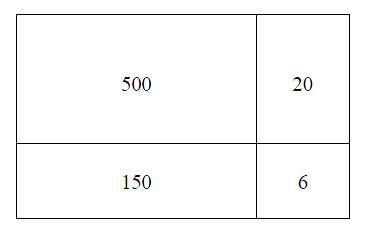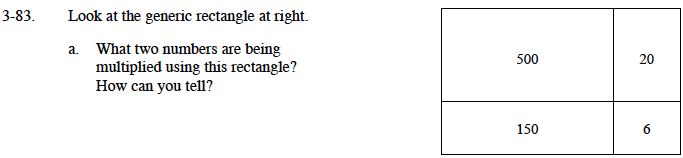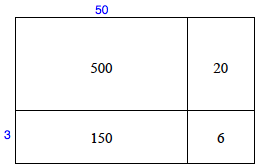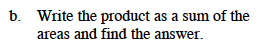### Home > MC1 > Chapter 3 > Lesson 3.3.3 > Problem3-83

3-83.
1. . Look at the generic rectangle at right. Homework Help ✎1. What two numbers are being multiplied using this rectangle? How can you tell?

2. Write the product as a sum of the areas and find the answer.Look back at problem 3-63 if you are not sure about using generic rectangles.

We can see that 3 is a factor of both 150 and 6, so we can put it on the diagram as shown.
Then we can see that 50 belongs at the top.Again, reference problem 3-63 as a guide for this problem. Remember that you are adding the smaller areas to find the total area.